﻿ Study on Voltage Characteristics of Distributed Power Supply Connected to Distribution NetworkPublications are Open
Access in this journal
Article Versions
Export Article
• Normal Style
• MLA Style
• APA Style
• Chicago Style
Research Article
Open Access Peer-reviewed

### Study on Voltage Characteristics of Distributed Power Supply Connected to Distribution Network

Zetian Zhong , Hang Zhang, Jiashu Wang, Gang Ma, Wei Qiu, Ying Wang
American Journal of Electrical and Electronic Engineering. 2019, 7(4), 99-104. DOI: 10.12691/ajeee-7-4-3
Received August 11, 2019; Revised September 29, 2019; Accepted October 18, 2019

### Abstract

With the rapid development of the world economy, the problem of energy crisis and environmental pollution is increasingly prominent. The traditional power generation mode not only consumes a lot of energy, but also causes a lot of pollution. Although distributed power supply brings benefits to people, due to the randomness and volatility of distributed power supply and the lack of unified grid-connected management system, the access of a large number of distributed power supply seriously affects the static voltage stability in the grid-connected area, resulting in voltage fluctuations, such as frequent high-voltage problems. Therefore, simulation research based on Matlab/Simulink platform is conducted in this paper. By changing the capacity and position of the distributed power supply connected to the distribution network, the optimal position and capacity of the distributed power supply connected to the distribution network are sought, so that the voltage on the distribution network is relatively stable at this time.

### 1. Introduction

Many scholars have studied on the problems caused by the connection of distributed power supply to distribution network. The law that various distributed power sources have different influences on various electrical indexes of the system with different access locations and capacities has been analyzed in Literature 1. The factors influencing voltage fluctuation of photovoltaic access to distribution network has been firstly analyzed from a theoretical perspective in Literature 2. Then from the point of view of short circuit capacity, the influence of ring network framework on voltage fluctuation is studied. Finally, PSCAD/EMTDC simulation software is used to build a complete photovoltaic power supply and IEEE13 node model, and corresponding examples are established to verify the above theory. A voltage characteristic analysis method based on the superposition principle has been proposed for the distribution network with photovoltaic access in Literature 3. Voltage drop at any point in the distribution network has been divided into voltage drop caused by the bus of the distribution network and voltage drop caused by photovoltaic power supply access, and studies have been carried out respectively. A dynamic reactive power distribution method has been proposed to solve the serious local voltage problem caused by large-scale access of distributed generation, and a reactive power optimization model of distribution network has been established in Literature 4. The original optimization problem has been simplified and transformed into a second-order cone programming model. According to the power model of wind farm, the influence of wind farm on node voltage when connected to simple power system has been analyzed in Literature 5, and when extended to complex power system, the fluctuation rule of node voltage has been revealed, the distribution characteristics of key nodes of voltage fluctuation has been summarized, which has provided basis for reactive power optimization.

The impact of distributed power supply on the voltage when it was connected to the distribution network has been described and studied above, but the impact caused by the changes of specific factors when the distributed power supply connected to the distribution network has not been studied in detail. By studying the problems caused by the access of the distributed power supply to the distribution network, the corresponding problems will be solved so that the distributed power supply can be better used by people. The impact of distributed power supply on distribution network voltage through the number of distributed power supply and the different access capacity and node of distributed power supply is studied and verified on Matlab/Simulink simulation software in this paper. The results show that as the capacity and node of distributed power supply access to distribution network are changed, they will have different impact on the voltage fluctuation of distribution network.

### 2. Distribution Network Modeling with Distributed Power Supply

Photovoltaic cells convert light energy directly into electrical energy through photoelectric or photochemical effects. The equivalent circuit diagram of photovoltaic cells is shown in Figure 1.

• Figure 1. Equivalent circuit of photovoltaic cell

The circuit consists of a series resistor RS, a shunt resistor Rsh and an optical drive current source. In the light condition, the PN junction of the photovoltaic cell will generate photocurrent Ipv . At this time, the current on the diode is ID. In order to make the accuracy meet the requirements of engineering analysis, the parallel resistance Rsh is added, which is used to simulate the leakage current caused by surface contamination or crystal defect. The series resistance RS represents the top surface resistance of the diffusion zone, the cell body resistance, the resistance between the upper and lower electrodes and the photovoltaic cell, and the resistance of the metal conductor 6. RL is the load resistance, IL is the load current, and VL is the load voltage.

As shown in the Figure 1: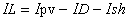(1)

Where, the photoelectric current source Ipv is: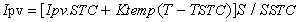(2)

The ID of current flowing through the diode is: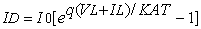(3)

The current Ish which is flowing through the shunt resistor Rsh is: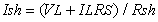(4)

Where, I0 is the reverse saturation current of the photovoltaic cell; q=1.6×10-19C; K=1.38×10-23J/K, which is called boltzmann constant; TSTC is the operating temperature of the photovoltaic cell in the standard state, which is the absolute temperature of the actual work of the photovoltaic cell, T= T +272K 7, A is the ideal factor of PN junction, S is the light intensity at the working point, and SSTC is the light intensity in the standard state.

### 3. Study on Voltage Characteristics of Distribution Network with Photovoltaic Power Supply Access

Preventing large voltage drop and voltage fluctuation when designing and managing distributed power grid is of great importance. When the photovoltaic power supply is connected to the distribution network, the original single power supply and radiant structure will become a multi-source complex network with small and medium-sized power supply, which will greatly change the original electrical state of the system and increase the difficulty in the planning, operation and maintenance of the distribution network.

In order to briefly analyze the impact on voltage of the distribution network with photovoltaic power supply access, an idealized distributed photovoltaic model is used in this paper, as shown in Figure 2.

• Figure 2. Simplifies the ideal distributed photovoltaic model

Where, the node whose voltage is U0 is the balance node, which remains constant voltage level ; UN is the rated voltage of the system.

The voltage drop on the R2+jX2 can be obtained from: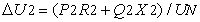(5)

Similarly, the voltage drop on R1+jX1 is: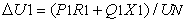(6)

At this time, the total voltage drop on the entire feeder is: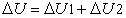(7)

The voltage of node 2 is: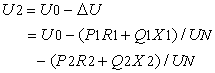(8)

If the active power and reactive power of the photovoltaic power supply input in node 1 are Pg and Qg, then the voltage on node 2: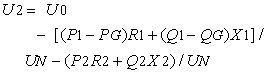(9)

If the active power and reactive power of the photovoltaic power supply input in node 2 are Pg and Qg, then the voltage on node 2: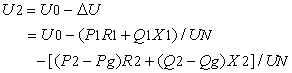(10)

It can be seen from the above that when the photovoltaic power supply is connected to the distribution network, the node voltage level of the distribution network will be greatly changed. In a word, when the photovoltaic power supply is connected, the node voltage of the distribution network will increase, and its influence on the node voltage will change with the power output and access position of the photovoltaic power supply. The photovoltaic power supply is usually equipped with reactive power compensation, so when it is connected to a node of the system, the active and reactive power flow through the node are reduced, so the maximum load that the line can bear also increases the input capacity of the power supply.

### 4. Simulation of Voltage Impact of Photovoltaic Power Supply Connected to IEEE33 Node Distribution Network on Distribution Network

In order to fully and concretely illustrate the influence of photovoltaic power supply access on the voltage of distribution network, the IEEE 33-node system is adopted as the simulation model to describe the variation degree of voltage before and after power flow calculation method. The IEEE 33-node system is a radial distribution network with a reference power of 10MVA and a voltage grade of 12.66kv. The total active load is 3715KW and the total reactive load is 2300KVar. The parameters of the line impedance and node load are shown in Literature 8.

The structure of IEEE 33 node network is shown in Figure 3.

There are many ways for photovoltaic power supply to be connected to IEEE 33-node distribution network. Generally speaking, it can be divided into single access or multiple photovoltaic power supply access. Under which the classification can be divided into a single node with different capacity accessed and different nodes with the same capacity accessed, etc., this article selects the node 3, 10, 17, 24, 27 and 30 as access points, in which three nodes 3, 10, 17 occupying the front, middle, back of the system mains respectively and three nodes 24,27,30 occupying three branches of the system respectively are included. The capacity shall be accessed according to 5%~30% of the total load capacity of the IEEE 33-node system (every 5% access).

• Figure 3. IEEE 33 node network structure diagram
4.1. Simulation of Voltage Impact of Single Photovoltaic Power Supply Connected to the Distribution Network

3, 10, 17, 24, 27 and 30 nodes are selected as the access points of a single distributed power supply, and 5%~30% of the total load of the system is accessed to each access point (5% each time), analyze the impact of single photovoltaic power supply on the system voltage with the same access location and different capacity.

When 5%~30% of the system capacity is connected to node 3, the system voltage distribution is shown in Figure 4.

• Figure 4. System voltage distribution diagram when node 3 is connected

When 5%~30% of the system capacity is connected to node 10, the system voltage distribution is shown in Figure 5.

• Figure 5. System voltage distribution diagram when node 10 is connected

When 5%~30% of the system capacity is connected to node 17, the system voltage distribution is shown in Figure 6.

• Figure 6. System voltage distribution diagram when node 17 is connected

When 5%~30% of the system capacity is connected to node 24, the system voltage distribution is shown in Figure 7.

• Figure 7. System voltage distribution diagram when node 24 is connected

When 5%~30% of the system capacity is connected to node 27, the system voltage distribution is shown in Figure 8.

• Figure 8. System voltage distribution diagram when node 27 is connected

When 5%~30% of the system capacity is connected to node 30, the system voltage distribution is shown in Figure 9.

• Figure 9. System voltage distribution diagram when node30 is connected

According to the above data and voltage distribution, the following conclusions can be drawn:

(1) When the photovoltaic power supply is connected to the distribution network, the original voltage amplitude level of the system will change greatly. Compared with the overall voltage level before photovoltaic power supply was connected to the relevant nodes of the distribution network, it increased and improved, and the voltage distribution curve is relatively stable.

(2)There is a linear relationship between the voltage amplitude of the distribution network and the output of the distributed power supply. The larger the output, the higher the voltage amplitude and the more stable the voltage distribution curve. Within the appropriate access range, the voltage level will not cause the risk of overvoltage and will be further and further away from the under voltage index.

(3) By comparing nodes 3, 10 and 17 with nodes 24, 27 and 30, it can be seen that photovoltaic power supply connected to the main line increases the voltage level to a greater extent and the voltage distribution is more stable than that connected to the branch line. However, according to relevant data, when the distributed power supply is connected to node 24 , with the increase of access capacity, its average voltage volatility does not always decrease, but increases after the access of 15% capacity. According to this, it can be found that when the distributed power supply is connected to the front-end branch of the system, the voltage of the nodes located in the same branch of the system can increase greatly, but the access contributes little to the voltage increase of other nodes, and can not improve the voltage stability of the system.

4.2. Simulation of Voltage Impact when Multiple Photovoltaic Power Sources are Connected to the Distribution Network

Select 3, 11, 19 and 4, 12, 20 and 5, 13, 21 as a group respectively to be multiple access points of photovoltaic power supply, stipulate that the access capacity of each group of nodes is 10%, 20% and 30% of the total load of the system, analyze the impact of multiple photovoltaic power supply on the system voltage with the same access position and different capacity.

Nodes 3, 11 and 19 are taken as the access points of the distributed power supply, and then select 10%, 20% and 30% of the system capacity for access. The voltage distribution of the system nodes is shown in Figure 10.

• Figure 10. Nodes 3, 11 and 19 are system voltage distributions of access points

Nodes 4, 12 and 20 are taken as access points of distributed power supply, and then select 10%, 20% and 30% of system capacity for access. The voltage distribution of system nodes is shown in Figure 11.

• Figure 11. Nodes 4, 12 and 20 are system voltage distributions of access points

Nodes 5, 13 and 21 are taken as access points of distributed power supply, and then select 10%, 20% and 30% of system capacity for access. The voltage distribution of system nodes is shown in Figure 12.

• Figure 12. Nodes 5, 13 and 21 are system voltage distributions of access points

### 5. Conclusion

(1) The access capacity of distributed power supply is an important factor that changes the voltage distribution and overall stability of the distribution network system.

(2) There is a linear relationship between the voltage amplitude of the distribution network and the output of the distributed power supply. The larger the output, the higher the amplitude, and the more stable the voltage.

(3) When a single photovoltaic power supply is connected to the distribution network with the capacity equals 20% of the system capacity, the voltage fluctuation is the minimum.

(4) When multiple photovoltaic power sources are connected to the distribution network, the larger the capacity of multiple photovoltaic power sources connected to the system, the smaller the voltage fluctuation will be.

(5) The nodes and capacity that are connected to the distribution network should be selected reasonably. Not all nodes and the increase of access capacity can reduce the voltage fluctuation and make the voltage of the distribution network stable.

### Acknowledgements

This research was supported by Postgraduate Research & Practice Innovation Program of Jiangsu Province (KYCX19_0809).

### References

  Can Wang, Qiuhong Wang,Jun Tang, et al. Influence of multiple distributed power supply access on network loss and voltage of distribution network. Journal of chongqing electric power college, 2018. In article  Yi Chen, Zhichen Liu,Jun Wu, Wei Chen, Yutian Xu. Research on the influence of distributed photovoltaic power supply on the voltage fluctuation of distribution network. Electric measurement and instrument, 2008, 55(14): 27-32. In article  Lin Zhu,Fu Dong Fu,Jianwei Zhai,Da Chen.Analysis on the influence of distributed photovoltaic power supply on node voltage of distribution network. Electric automation, 2008, 40(06):74-77. In article  Shaohua L , Jiekang W U , Chao M O, et al. Dynamic Partition and Optimization Method for Reactive Power of Distribution Networks With Distributed Generation Based on Second-Order Cone Programming. Power System Technology, 2018. In article  Yixi Chen, Guchao Xu, Gang Ma, Feng Li, Rong Ju. Research on the Voltage Fluctuation Rules of Power System Containing Wind Farms[C]//Proceedings of 2017 2nd Asia Conference on Power and Electrical Engineering(ACPEE 2017). Shanghai, China: IOP, 2017, 012019. (EI,CPCI-S). In article View Article  Mash.J. Bollen, Van An Hassan, et al. Distributed generation access to power system. China machine press, 2015. In article  Shihong Shi, Fang Guo. Application and influence of photovoltaic power generation in 500kV substation. China electric power, 2014, 47(12): 105-109. In article  Shouxiang Wang,Chengshan Wang. Analysis of modern power distribution system. Higher education press, 2007. In article

Published with license by Science and Education Publishing, Copyright © 2019 Zetian Zhong, Hang Zhang, Jiashu Wang, Gang Ma, Wei Qiu and Ying WangThis work is licensed under a Creative Commons Attribution 4.0 International License. To view a copy of this license, visit http://creativecommons.org/licenses/by/4.0/